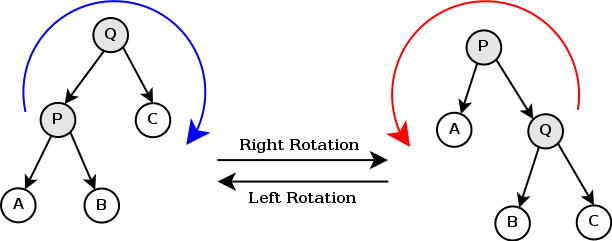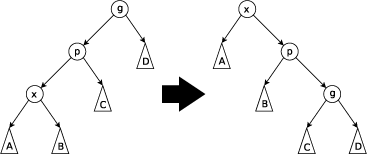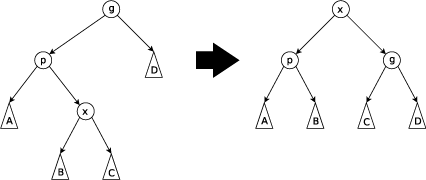# 从 BST 到 Splay

### 从 BST 到 Splay树

BST (二叉排序树)、AVL 树、替罪羊树、Splay伸展树，红黑树，B树 等

BST (二叉排序树)

$如果左子树不为空，则左子树上所有结点的值均小于根结点的值。$

$如果右子树不为空，则右子树上所有结点的值均大于根结点的值。$

$左、右子树也分别为二叉排序树$

AVL

$LL：LeftLeft$，也称“左左”。插入或删除一个节点后，根节点的左孩子（Left Child）的左孩子（Left Child）还有非空节点，导致根节点的左子树高度比右子树高度高2，AVL树失去平衡。

$RR：RightRight$，也称“右右”。插入或删除一个节点后，根节点的右孩子（Right Child）的右孩子（Right Child）还有非空节点，导致根节点的右子树高度比左子树高度高2，AVL树失去平衡。

$LR：LeftRight$，也称“左右”。插入或删除一个节点后，根节点的左孩子（Left Child）的右孩子（Right Child）还有非空节点，导致根节点的左子树高度比右子树高度高2，AVL树失去平衡。

$RL：RightLeft$，也称“右左”。插入或删除一个节点后，根节点的右孩子（Right Child）的左孩子（Left Child）还有非空节点，导致根节点的右子树高度比左子树高度高2，AVL树失去平衡$图片来自wiki$

//right右旋为1，left左旋为0
void rotate(ll x,int op){
//1)找父亲
ll y = f[x], z = f[y];
//ch[x][] 表示左0,右1儿子结点
//2)右旋右挂左，左旋左挂右
ch[y][op^1] = ch[x][op];
if(!ch[x][op]) f[ch[x][op]] = y;
f[x] = z,f[y] = x;
//如果祖先结点存在，根据y为z的左右孩子位置，用于x来替换
if(!z) ch[z][ch[z]==y] = x;
ch[x][op] = y;
}



Splay

splay(x) = while(f[x]!=root) rotate(x);


$Zig-Zig$当p不是根节点，且x和p同为左孩子或右孩子时进行Zig-Zig操作。



$Zig - Zag$当p不是根节点，且x和p不同为左孩子或右孩子时，进行Zig-Zag操作。


void splay(ll x,ll root){
while(f[x]!=root){
ll y = f[x],z = f[y];
//单旋情况
if(z == root){
if(ch[y] == x) rotate(x,0);//x为右子则左旋
else rotate(x,1);//否则右旋
}
//双旋情况
else{
if(ch[z] == y){
if(ch[y] == x) rotate(y,1), rotate(x,1); // LL
else rotate(x,0), rotate(x,1);//LR
}else{
if(ch[y] == x) rotate(y,0), rotate(x,0);//RR
else rotate(x,1), rotate(x,0);//RL
}
}
}
}


void find(ll x){
ll u = root;
if(!u) return;//空树
while(ch[u][x > w[u]] && x != w[u])//当存在儿子并且当前位置的值不等于x
u = ch[u][x > w[u]];
splay(u,0);//将找到的 x 向上旋转
}


void insert(ll x){
ll u = root,fu = 0;
//u存在且没有查找到x
while(u && w[u] != x){
fu = u;
u = ch[u][x > w[u]];
}
if(u) num[u]++;//则增加一个这样的数
else{
u = ++tot;//新增结点
//父节点非根
if(fu) ch[fu][x > w[fu]] = u;
ch[u] = ch[u] = 0;//不存在儿子
f[tot] = fu;//父节点
w[tot] = x;//结点值
num[tot] = 1;//含有此数的个数
siz[tot] = 1;//含有不同个数值
}
splay(u,0);//旋转并保证结构平衡
}


ll get_nex(ll x,ll nx){
find(x);
ll u = root;//根节点
if(w[u] > x && nx) return u;//如果当前节点的值大于x并且要查找的是后继
if(w[u] < x && !nx) return u;
u = ch[u][nx]; //查找后继的话在右儿子上找，前驱在左儿子上找
while(ch[u][nx^1]) u = ch[u][nx^1];//要反着跳转，否则会越来越大（越来越小）
return u;
}


void delete(ll x){
ll las = get_nex(x,0);
ll nex = get_nex(x,1);
splay(las,0),splay(nex,las);
ll del = ch[nex];
//删除值个数超过1个
if( num[del] > 1){
num[del]--;
splay(del,0);
}else{
ch[nex] = 0;//此节点直接删除
}
}


ll kth(ll x){
ll u = root;
//如果树上没有这么多的数
if(size[u] < x) return 0;
while(1){
ll y= ch[u];//左儿子
if(x>size[y]+num[u])
//如果排名比左儿子的大小和当前节点的数量要大
{
x-=size[y]+num[u];//数量减少
u= ch[u];//那么当前排名的数一定在右儿子上找
}
else//否则的话在当前节点或者左儿子上查找
if(size[y]>=x)//左儿子的节点数足够
u=y;//在左儿子上继续找
else//否则就是在当前根节点上
return w[u];
}
}


struct Splay
{
#define maxn (int)1e5+5
//个数，大小,权值
int num[maxn],siz[maxn]; ll w[maxn];
//父亲，孩子
int f[maxn],ch[maxn];
// 下标，根节点
int tot,root;

void splay(int x,int root){
while(f[x]!=root){
int y = f[x],z = f[y];
//单旋情况
if(z == root){
if(ch[y] == x) rotate(x,0);//x为右子则左旋
else rotate(x,1);//否则右旋
}
//双旋情况
else{
if(ch[z] == y){
if(ch[y] == x) rotate(y,1), rotate(x,1); // LL
else rotate(x,0), rotate(x,1);//LR
}else{
if(ch[y] == x) rotate(y,0), rotate(x,0);//RR
else rotate(x,1), rotate(x,0);//RL
}
}
}
}

void find(int x){
int u = root;
if(!u) return;//空树
while(ch[u][x > w[u]] && x != w[u])//当存在儿子并且当前位置的值不等于x
u = ch[u][x > w[u]];
splay(u,0);//将找到的 x 向上旋转
}

void insert(int x){
int u = root,fu = 0;
//u存在且没有查找到x
while(u && w[u] != x){
fu = u;
u = ch[u][x > w[u]];
}
if(u) num[u]++;//则增加一个这样的数
else{
u = ++tot;//新增结点
//父节点非根
if(fu) ch[fu][x > w[fu]] = u;
ch[u] = ch[u] = 0;//不存在儿子
f[tot] = fu;//父节点
w[tot] = x;//结点值
num[tot] = 1;//含有此数的个数
siz[tot] = 1;//含有不同个数值
}
splay(u,0);//旋转并保证结构平衡
}

int get_nex(int x,int nx){
find(x);
int u = root;//根节点
if(w[u] > x && nx) return u;//如果当前节点的值大于x并且要查找的是后继
if(w[u] < x && !nx) return u;
u = ch[u][nx]; //查找后继的话在右儿子上找，前驱在左儿子上找
while(ch[u][nx^1]) u = ch[u][nx^1];//要反着跳转，否则会越来越大（越来越小）
return u;
}

void delete(int x){
int las = get_nex(x,0);
int nex = get_nex(x,1);
splay(las,0),splay(nex,las);
int del = ch[nex];
//删除值个数超过1个
if( num[del] > 1){
num[del]--;
splay(del,0);
}else{
ch[nex] = 0;//此节点直接删除
}
}

ll kth(int x){
int u = root;
//如果树上没有这么多的数
if(size[u] < x) return 0;
while(1){
int y= ch[u];//左儿子
if(x>size[y]+num[u])
//如果排名比左儿子的大小和当前节点的数量要大
{
x-=size[y]+num[u];//数量减少
u= ch[u];//那么当前排名的数一定在右儿子上找
}
else{
//否则的话在当前节点或者左儿子上查找
//左儿子的节点数足够
if(size[y]>=x){
u=y;//在左儿子上继续找
}
else{
//否则就是在当前根节点上
return w[u];
}
}
}
}
}splay;

posted @ 2020-05-15 22:15  Tianwell  阅读(201)  评论(0编辑  收藏  举报